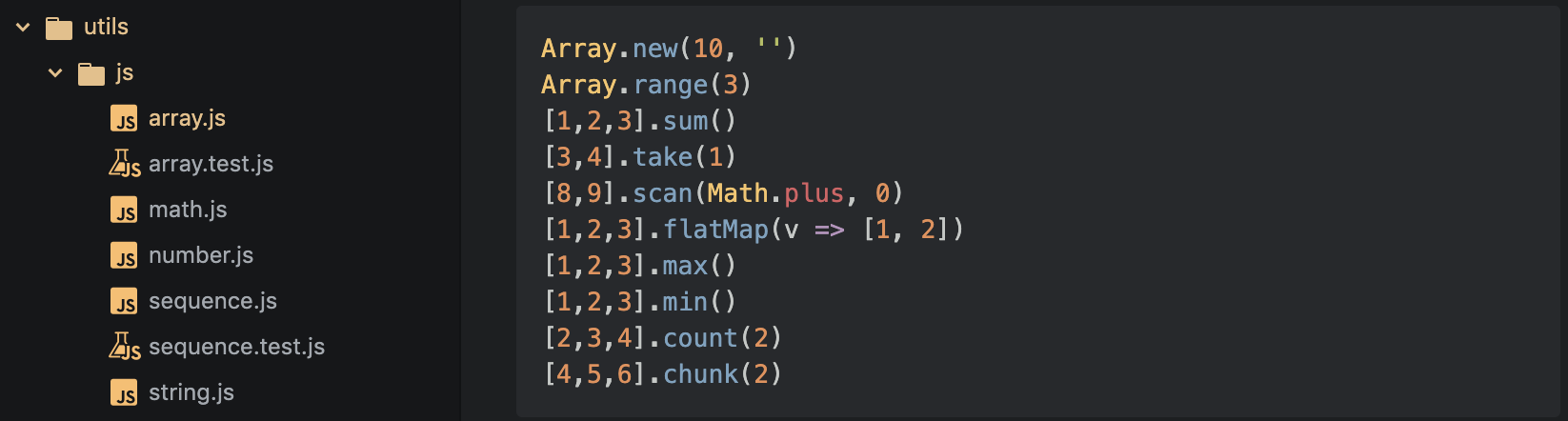# Advent of Code 2015 Javascript Array UtilityJavascript Array Utility

When I am working for competition, I find that I always need to write some utility function for to simplify the workflow. This is especially true after I learned a few tricks from and . And I realize that the language does not matter too much for simple problems. What matters more is the way of drafting the workflow and writing functions.

# Functions

`import _ from 'lodash'`

I used as my baseline, but you can replace it with any other similar libraries. The main reason to use it here is to save time to support various chaining operations for .

## New

`// NewArray.new = function (n, v) {  return new Array(n).fill(v)}// ExampleArray.new(3, ' ') -> [' ', ' ', ' ']`

## Range

`// RangeArray.range = function (start, end) {  return _.range(start, end)}// ExampleArray.range(3) -> [0,1,2]Array.range(1,3) -> [1,2]`

I found pretty useful, and in other languages, you can simply do .

## Then

`// ApplyArray.prototype.then = function (fn) {  return fn(this)}// Examplefn = v => 1[0,1].then(fn) -> 1`

This is a quick function which can be used to start/continue the chaining. It simply returns the invoked function result, similar in the handling. Semantic wise, it fits perfectly into the workflow.

## Sum

`// Sumconst sum = d => {  if (d) return (acc, s) => acc + d(s)  return (acc, s) => acc + s}Array.prototype.sum = function (predicate) {  return _.reduce(this, sum(predicate), 0)}// Example[1,2].sum() -> 3[1,2].sum(v => v*v) -> 5`

Sum is another useful one, there’s absolutely no need to write a loop for it. Sum is implemented via a operation.

## Count

`// CountArray.prototype.count = function (fn) {  if (typeof fn === 'function') return this.filter(fn).length  return this.filter(v => v === fn).length}// Example[1,2,1,3].count(1) -> 2[1,2].count(v => v == 1) -> 1`

Once again, this is another function that absolutely you don’t want to write it again. Very handy and make sense, all language should just support it by native.

## Scan

`// Scan// https://github.com/lodash/lodash/issues/1684function scan(array, fn, seed, thisArg) {  if (thisArg) fn = fn.bind(thisArg);  var size = array.length,    i,    accumulator,    results;  if (seed !== void 0) {    results = Array(size + 1);    accumulator = seed;    for (i = 0; i < size; ++i) {      results[i] = accumulator;      accumulator = fn(accumulator, array[i]);    }    results[i] = accumulator;  } else {    results = Array(size);    accumulator = results = array;    for (i = 1; i < size; ++i) {      results[i] = accumulator = fn(accumulator, array[i]);    }  }  return results;}Array.prototype.scan = function (fn, seed) {  return scan(this, fn, seed)}// Example[1,2].scan(Math.plus, 0) -> [0,1,2]`

Scan is a new addition to functional programming community and it’s not included by default. But I think it’s a must-have, because it meets the gap between and . If you rely on any initial condition and care about each intermediate result, this is the only way to go. Scan returns the initial condition as the first element.

## Flatmap

`// FlatmapArray.prototype.flatMap = function (fn) {  return _.flatMap(this, fn)}// Example[0,1].flatmap(v => [1, 2]) -> [1,2,1,2]`

Flatmap is another very useful workflow operation. It combines all the results of array and then flatten them to the one array. You can think of it as distributing work to other units and then gathering them back. Very powerful indeed.

## Rest

`// TransformArray.prototype.transform = function (iter, acc) {  return _.transform(this, iter, acc)}// UniqeArray.prototype.uniqBy = function (iteratee) {  return _.uniqBy(this, iteratee)}// TakeArray.prototype.take = function (n) {  return _.take(this, n)}// TakeWhileArray.prototype.takeWhile = function (predicate) {  return _.takeWhile(this, predicate)}// RemoveArray.prototype.remove = function () {  var what, a = arguments, L = a.length, ax;  while (L && this.length) {    what = a[--L];    while ((ax = this.indexOf(what)) !== -1) {      this.splice(ax, 1);    }  }  return this;}Array.prototype.max = function () {  return Math.max(...this)}Array.prototype.min = function () {  return Math.min(...this)}Array.prototype.chunk = function (n) {  return _.chunk(this, n)}// [0, 1, 2, 3] => [0, 2], [1, 3]Array.prototype.chunkMod = function (n) {  return _.range(n).map(i => this    .filter((_, j) => j % n == i)  )}`

# Highlights

• How to use small utility function to make your life one magnitude easier?

For Complete source code, please visit AoC 2015 Javascript.